Area between curve and line

Chapter 8 Class 12 Application of Integrals
Concept wise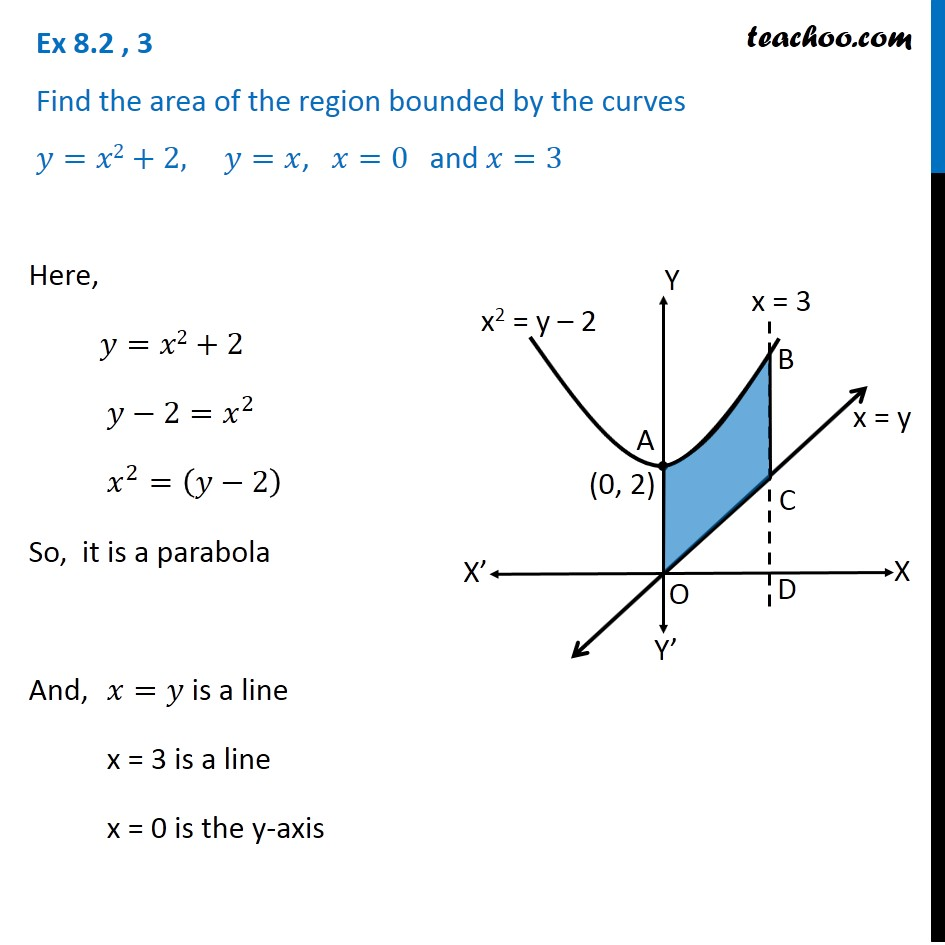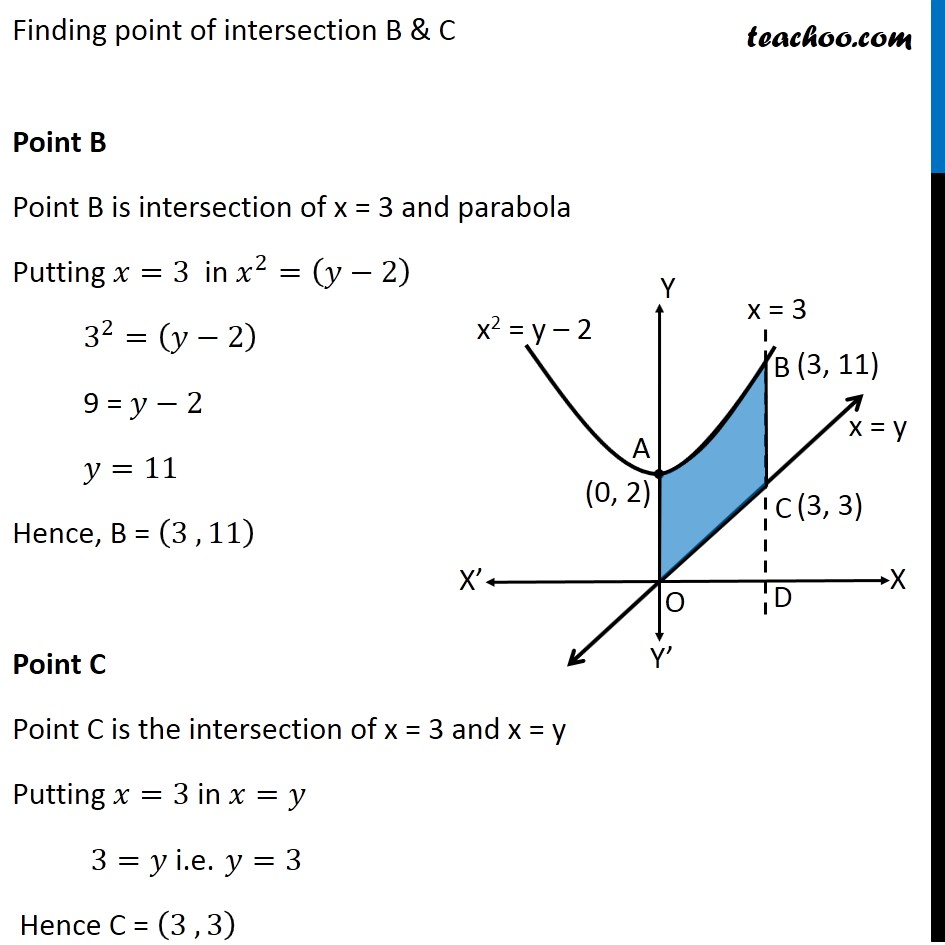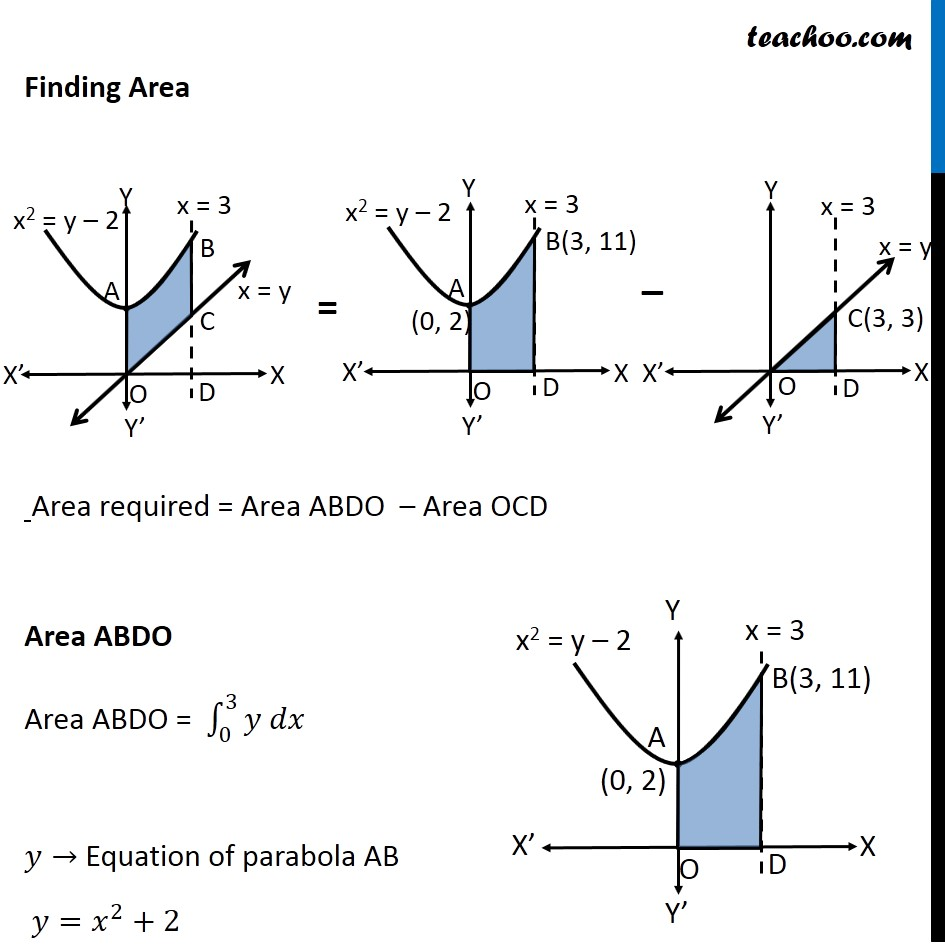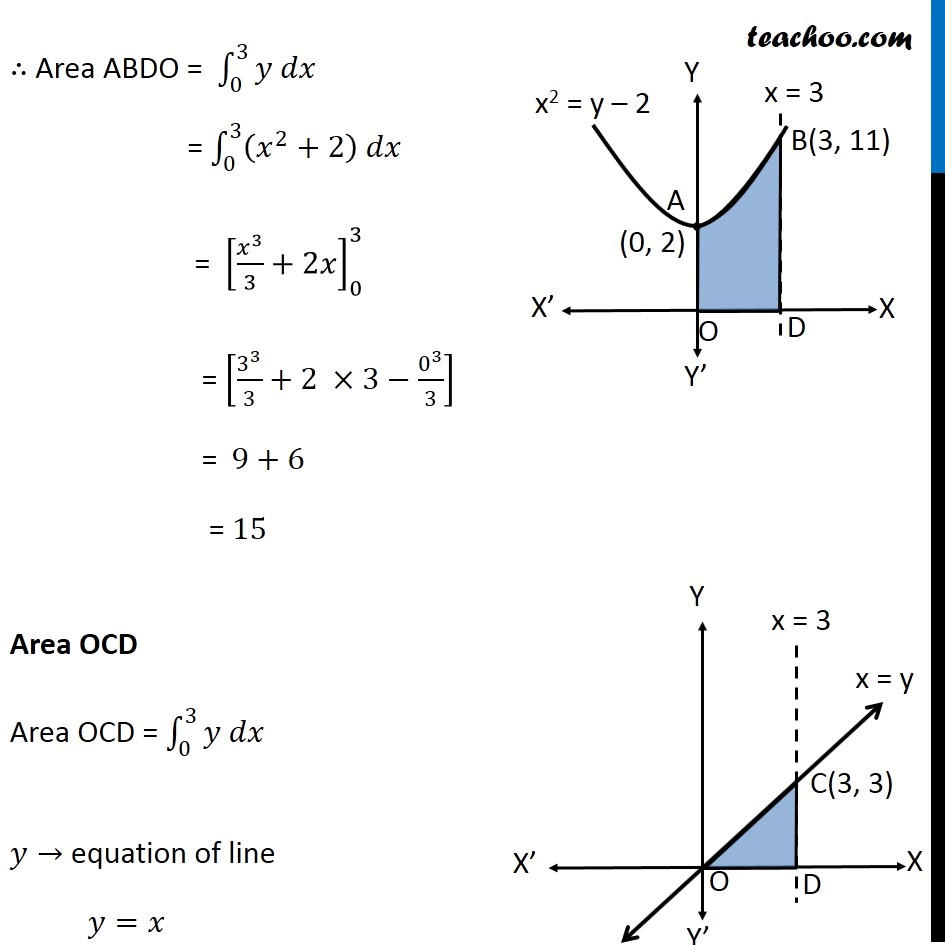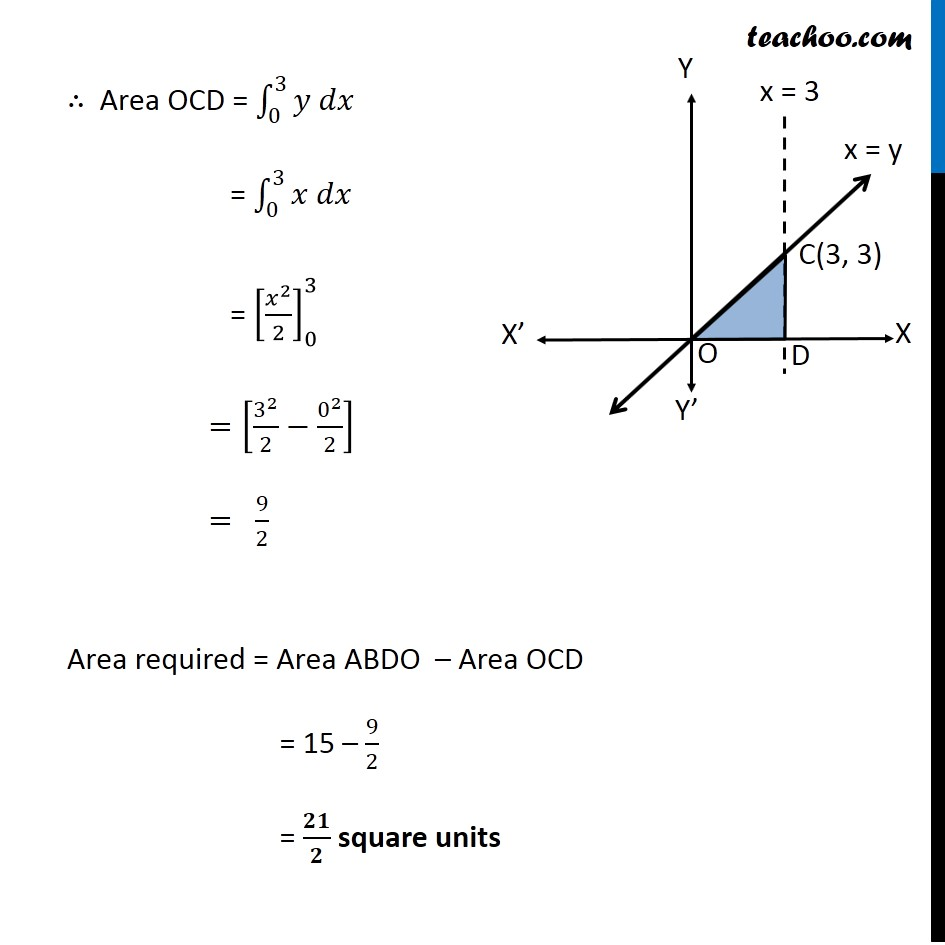Introducing your new favourite teacher - Teachoo Black, at only ₹83 per month

### Transcript

Ex 8.2 , 3 Find the area of the region bounded by the curves 𝑦=𝑥2+2, 𝑦=𝑥, 𝑥=0 and 𝑥=3 Here, 𝑦=𝑥2+2 𝑦−2=𝑥^2 𝑥^2=(𝑦−2) So, it is a parabola And, 𝑥=𝑦 is a line x = 3 is a line x = 0 is the y-axis Finding point of intersection B & C Point B Point B is intersection of x = 3 and parabola Putting 𝑥=3 in 𝑥^2=(𝑦−2) 3^2=(𝑦−2) 9 = 𝑦−2 𝑦=11 Hence, B = (3 , 11) Point C Point C is the intersection of x = 3 and x = y Putting 𝑥=3 in 𝑥=𝑦 3=𝑦 i.e. 𝑦=3 Hence C = (3 , 3) Finding Area Area required = Area ABDO – Area OCD Area ABDO Area ABDO = ∫_0^3▒〖𝑦 𝑑𝑥〗 𝑦→ Equation of parabola AB 𝑦=𝑥^2+2 ∴ Area ABDO = ∫_0^3▒〖𝑦 𝑑𝑥〗 = ∫_0^3▒〖(𝑥^2+2) 𝑑𝑥〗 = [𝑥^3/3+2𝑥]_0^3 = [3^3/3+2 ×3−0^3/3] = 9+6 = 15 Area OCD Area OCD = ∫_0^3▒〖𝑦 𝑑𝑥〗 𝑦→ equation of line 𝑦=𝑥 ∴ Area OCD = ∫_0^3▒〖𝑦 𝑑𝑥〗 = ∫_0^3▒〖𝑥 𝑑𝑥〗 = [𝑥^2/2]_0^3 =[3^2/2−0^2/2] = 9/2 Area required = Area ABDO – Area OCD = 15 – 9/2 = 𝟐𝟏/𝟐 square units